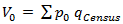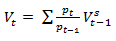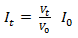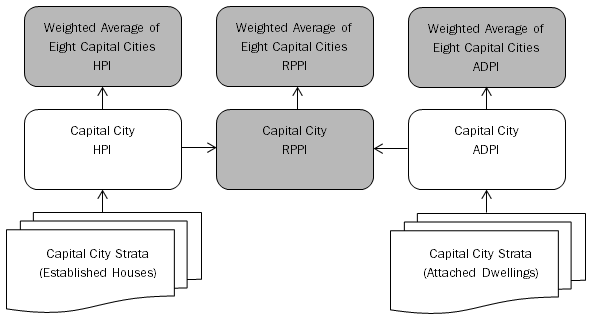6464.0 - Residential Property Price Indexes: Concepts, Sources and Methods, 2018
Latest ISSUE Released at 11:30 AM (CANBERRA TIME) 19/03/2019
Page tools:Print All

CALCULATION IN PRACTICE

INTRODUCTION

8.1 This section will expand on information provided in previous sections to describe how the indexes are calculated in practice.

STRATIFICATION METHODOLOGY

8.2 As outlined in the previous sections, the approach adopted by the ABS to control for quality and compositional change in measuring price movements is stratification. The allocation of suburbs to strata (the building blocks of the indexes) in each city is determined by this method.

8.3 Following the 2007 index review, the optimum stratification was defined as one which maximised the homogeneity of the suburbs in a stratum, while also maximising the number of price observations each quarter. That is, reducing the number of strata in a city would be expected to increase the number of price observations in most strata, producing more robust stratum medians from which to derive price relatives. However, it could also have the effect of creating less homogenous strata.

8.4 The resultant stratification was simplified to stratify suburbs according to long-term median price and SEIFA (footnote 14).

8.5 The recommendations of the 2007 review were implemented with the introduction of Series 2 in the December quarter 2008 (footnote 15).

8.6 The following table shows the number of strata currently used for each city (Series 4), compared to the previous series (Series 3).

 TABLE 1 Number of StrataSeries 4 (from September quarter 2018 onwards) Series 3 (from March quarter 2013 onwards)HPI ADPI HPI ADPISydney 21 13 23 12 Melbourne 16 14 19 13 Brisbane 16 9 13 9 Adelaide 13 11 13 8 Perth 13 12 13 10 Hobart 8 6 8 4 Darwin 5 5 6 7 Canberra 8 8 10 8

IMPUTATION AND EDITING

8.7 As outlined in Scope and Coverage, the stratification methodology chosen aims to produce strata which maximise both the homogeneity of the price-determining characteristics of dwellings in a stratum, and the number of price observations that could be expected each period, in order to calculate a reliable median price.

8.8 However, in practice, some suburbs (the building blocks of the indexes) are so unique that to include them with other suburbs would produce strata which do not meet homogeneity requirements. Therefore, some strata are formed from one or very few suburbs, which means that the number of price observations available each quarter is extremely low, or sometimes nil.

8.9 The price movements of strata with 'missing' observations in a particular quarter are therefore derived using an appropriate imputation method during the editing process.

8.10 Duplicate records within the residential property sales dataset are also identified in the editing stage. In addition, some records received may have a very high or low price which is the result of a data entry error; the duplicate and incorrect records do not contribute to median price calculations.

CALCULATING WEIGHTS

8.11 As discussed in Weights and their Sources, the weights used in calculating the indexes are value weights. The quantities underpinning these weights are obtained from the latest Census of Population and Housing. However, the price reference period of the property price indexes is usually later than the period from which the quantities are obtained. For example, the current price reference period of the indexes is the September quarter 2018, however the latest Census was in August 2016.

8.12 Rather than calculating value weights with prices and quantities from this earlier period, it is common to price update (revalue) the quantities from the weight reference period to the price levels of the price reference period and link period (footnote 16) (where the new index series is to be linked to an existing series). The effect is to update the value of the underlying quantities to align with the most recent set of price information.

8.13 For the HPI and ADPI, price updated values of the dwelling stock are determined by multiplying quantities from the weight reference period by prices from the price reference period for each stratum.

8.14 Strata quantities are dwelling counts obtained from 2016 Census data.

8.15 Strata prices are derived as the 'mean-adjusted median' for the price reference period (currently September quarter 2018). This measure is calculated by finding the ratio of the mean and median for the four consecutive quarters up to and including the link period, and then averaging these ratios. This average ratio is applied to the link period median with the intention of deriving a more robust 'mean' price for the strata than is possible by calculating a mean price for one quarter (which is influenced by any unusual transactions).

8.16 The resulting value aggregate (v0) is then expressed in terms of prices (p0) from the price reference period (period 0) and quantities (qCensus) from the weight reference period. Value aggregates for the capital cities are simply the sum of the strata value aggregates. This can be expressed through the following formula:(Equation 1)

8.17 The calculation of capital city index numbers occurs in two stages. Firstly, a price relative is calculated for each stratum. This price relative is then used to calculate a current period (period t) value of the dwelling stock for each stratum. From there, the values from the strata are aggregated, and published index numbers are subsequently derived from these value aggregates. Therefore, the movement in the capital city index is a weighted average of the movements of each stratum.

8.18 The price relative for each stratum is the ratio of the median price in the current period (pt) to the previous period (pt-1). The value aggregate (vt) is therefore calculated as:(Equation 2)

8.19 The index numbers are calculated as the ratio of the value aggregate in the current period to the value aggregate in the price reference period multiplied by the index number in the price reference period (I0). For example, for the current period t, and price reference period 0 the index number for time t (It) is calculated as:(Equation 3)

8.20 The process can be illustrated by the example in Tables 2 and 3 which show the index calculation for a city which is made up of five strata. In this example, the first step is to calculate the price movement for each stratum i.e. the price relative. The next step is to calculate a current period value aggregate for each stratum by using the price relative to inflate or deflate the previous period value. The strata values can be aggregated to produce values for the city. The final step is to produce a current period price index by dividing the current period value by the price reference period value and then multiplying this by the price reference period index number. This example demonstrates that the movement in the aggregate index is determined not just by the price movements, but also by the weights. Strata 1 shows a very large price fall, however its impact on the overall index movement reflects its relatively low weight.

 TABLE 2 Calculating Price RelativesPrevious Period (pt-1) Current Period (pt) Price Relative (pt / pt-1) Strata 1\$ 1 500 000 \$ 1 260 000 0.840 Strata 2\$ 800 000 \$ 800 000 1.000 Strata 3\$ 500 000 \$ 505 000 1.010 Strata 4\$ 400 000 \$ 412 000 1.030 Strata 5\$ 300 000 \$ 315 000 1.050

 TABLE 3 Calculating Index NumbersPrice Reference Period Previous Period Current Period % change VALUE AGGREGATES (\$' 000) Strata 1 600 000 650 000 546 000 –16.0 Strata 2 8 000 000 7 500 000 7 500 000 0.0 Strata 3 15 000 000 16 000 000 16 160 000 1.0 Strata 4 15 000 000 17 500 000 18 025 000 3.0 Strata 5 2 000 000 3 200 000 3 360 000 5.0 City A 40 600 000 44 850 000 45 591 000 1.7 INDEX NUMBERS (no.) Strata 1 105.0 113.8 95.6 –16.0 Strata 2 105.0 98.4 98.4 0.0 Strata 3 94.0 100.3 101.3 1.0 Strata 4 91.0 106.2 109.4 3.0 Strata 5 96.0 153.6 161.3 5.0 City A 93.0 102.7 104.4 1.7

PRICE INDEXES FOR HIGHER LEVEL COMPONENTS

8.21 The below diagram shows the relationship between the strata and published level indexes: capital city HPI and ADPI, the RPPI and the weighted eight capital city indexes.

8.22 Shaded boxes represent the higher level component indexes which are calculated by combining the value aggregates of published indexes.

Figure 1 Structure of the RPPI, HPI and ADPI8.23 Combining value aggregates from the capital city indexes allows for the calculation of higher level price indexes, for example the weighted average of eight capital cities (which is an aggregate of each capital city index) and the RPPI (which is an aggregation of the HPI and ADPI).

8.24 Aggregation of these higher level price indexes occur much the same way as in Table 3, except at a higher level in the index structure so rather than strata value aggregates being combined it is capital city index value aggregates that are being combined. A simplified example is below.

 TABLE 4 Calculating Higher Level IndexesPrice Reference Period Previous Period Current Period % change VALUE AGGREGATES (\$' 000) City A HPI 40 600 000 44 850 000 45 591 000 1.7 City B HPI 30 200 000 32 500 000 34 000 000 4.6 Weighted HPI 70 800 000 77 350 000 79 591 000 2.9 INDEX NUMBERS (no.) City A HPI 93.0 102.7 104.4 1.7 City B HPI 95.0 102.2 106.9 4.6 Weighted HPI 94.0 102.7 105.7 2.9

8.25 Users should note that prior to March quarter 2008, the RPPI was calculated as a fixed weighted average of the HPI and ADPI (see the June quarter 2012 issue of cat. no. 6416.0 for further information).

REVISIONS

8.26 The residential property price indexes are considered final. Revisions would only occur in exceptional circumstances.

ROUNDING CONVENTIONS

8.27 To ensure consistency in the application of data produced from ABS price indexes, it is necessary for the ABS to adopt a set of consistent rounding conventions or rules for the calculation and presentation of data. The conventions strike a balance between maximising the usefulness of the data for analytical purposes and retaining a sense of the underlying precision of the estimates. These conventions need to be taken into account when using price index data for analytical or other special purposes.

8.28
Index numbers are always published to an index reference period of 100.0. Index numbers and percentage changes are always published to one decimal place, with the percentage changes being calculated from the rounded index numbers. Index numbers for periods longer than a single quarter (e.g. for financial years) are calculated as the simple arithmetic average of the relevant rounded quarterly index numbers. Percentage changes between these periods are calculated from the rounded average index numbers.

Footnotes:
14 See Census of Population and Housing: Socio-Economic Indexes for Areas (SEIFA), Australia, 2016 (cat. no. 2033.0.55.001). <back
15 Information on the stratification methodology used is available from Information Paper: Renovating the Established House Price Index, Nov 2005 (cat. no. 6417.0). <back
16 For the property price indexes, the link period and price reference period are always the same due to the way strata are used in the indexes. Other price indexes may have price reference periods that differ from the link period. Further information can be obtained from the Concepts, Sources and Methods publications for the relevant indexes. <back